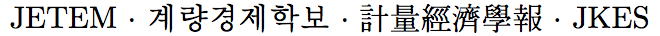Journal of Economic Theory and Econometrics: Journal of the Korean Econometric Society
Home

Archive

Search

Journal of Economic Theory and EconometricsJournal of the Korean Econometric Society

Estimation of Regime-Switching Continuous-Time Stochastic Volatility Models Using KOSPI 200Vol.30, No.1, March 2019, 59–95

English Version |  Korean Version
• Seungmoon Choi   (University of Seoul)Abstract

This article estimates regime-switching continuous-time stochastic volatility models using daily KOSPI 200. We consider single regime Heston, GARCH, and CEV stochastic volatility models and 6 regime-switching stochastic volatility models which have two different regimes $L$ and $H$. We employ Hamilton algorithm (Hamilton (1989)) to compute the log-likelihood and to apply MLE. Because the true transition probability density functions (TPDFs) of our stochastic volatility models are unknown, we use Ait-Sahalia (2008) and Choi (2015b) to obtain closed-form approximate TPDF. The regime-switching CEV model where the transition probability is allowed to vary over time has been found to be the best to explain the movements of KOSPI 200. Regime $L$ has a stronger leverage effect than regime $H$. Comparing to regime $L$, the volatility variable tends to revert to its long-run mean level more rapidly, the volatility of volatility variable is greater, the probability of staying in the same regime $H$ in the next period is bigger in regime $H$. And the transition probability varies with time depending on the stock price rather than the volatility. When the probabilities of regime $H$ are high we could identify various economic and political events between South Korea and North Korea or inside or outside South Korea that could have affected Korean stock market.

Keywords
KOSPI 200, Continuous-Time Stochastic Volatility Model, Regime-Switching, Maximum Likelihood Estimation

JEL classification codes
C22, C51, C58